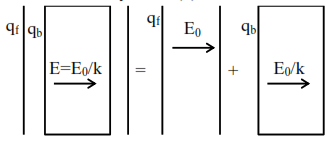# Solve this

Question:

If $\mathrm{q}_{\mathrm{f}}$ is the free charge on the capacitor plates and $\mathrm{q}_{\mathrm{b}}$ is the bound charge on the dielectric slab of dielectric constant $k$ placed between the capacitor plates, then bound charge $\mathrm{q}_{\mathrm{b}}$ can be expressed as :

1. $\mathrm{q}_{\mathrm{b}}=\mathrm{q}_{\mathrm{f}}\left(1-\frac{1}{\sqrt{\mathrm{k}}}\right)$

2. $\mathrm{q}_{\mathrm{b}}=\mathrm{q}_{\mathrm{f}}\left(1-\frac{1}{\mathrm{k}}\right)$

3. $\mathrm{q}_{\mathrm{b}}=\mathrm{q}_{\mathrm{f}}\left(1+\frac{1}{\sqrt{\mathrm{k}}}\right)$

4. $\mathrm{q}_{\mathrm{b}}=\mathrm{q}_{\mathrm{f}}\left(1+\frac{1}{\mathrm{k}}\right)$

Correct Option: , 2

Solution:When a dielectric is inserted in a capacitor

Due to free charge $\overrightarrow{\mathrm{E}}=\overrightarrow{\mathrm{E}}_{0}$ only

After dielectric $\mathrm{E}^{\prime}=\frac{\mathrm{E}_{0}}{\mathrm{k}}$

$\mathrm{q}_{\mathrm{B}}=\mathrm{q}_{\mathrm{f}}\left(1-\frac{1}{\mathrm{k}}\right)$